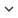ENGArticle
Article 348
Author/s
• Constantino A. García, Abraham Otero, Paulo Félix, Jesús Presedo, and David G. Márquez
DOI
Source
• Physical Review E, 2017 - Q1

# Nonparametric estimation of stochastic differential equations with sparse Gaussian processes

The application of stochastic differential equations (SDEs) to the analysis of temporal data has attracted increasing attention, due to their ability to describe complex dynamics with physically interpretable equations. In this paper, we introduce a nonparametric method for estimating the drift and diffusion terms of SDEs from a densely observed discrete time series. The use of Gaussian processes as priors permits working directly in a function-space view and thus the inference takes place directly in this space. To cope with the computational complexity that requires the use of Gaussian processes, a sparse Gaussian process approximation is provided. This approximation permits the efficient computation of predictions for the drift and diffusion terms by using a distribution over a small subset of pseudosamples. The proposed method has been validated using both simulated data and real data from economy and paleoclimatology. The application of the method to real data demonstrates its ability to capture the behavior of complex systems.
Keywords: Stochastic Differential Equation, Langevin Equation, Gaussian Processes, Sparse Gaussian Processes, Variational Inference